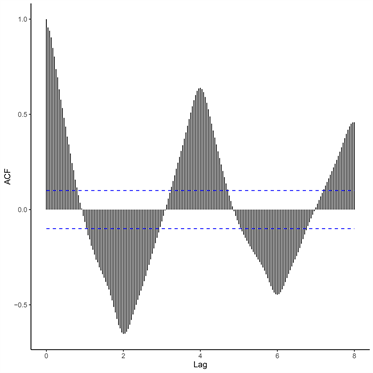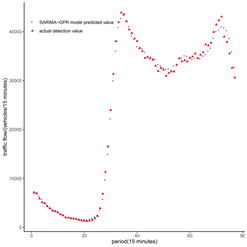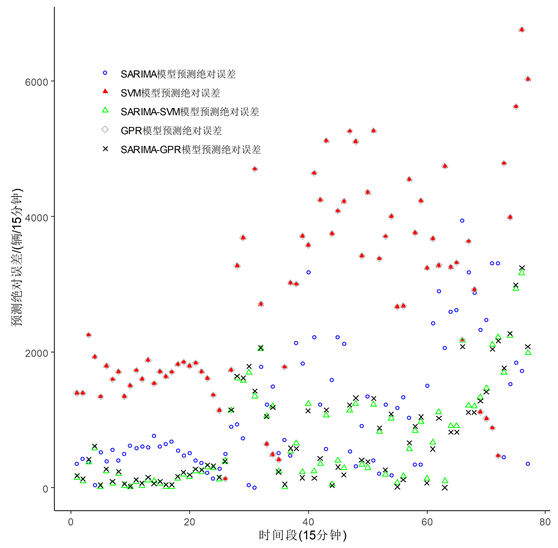#### 期刊菜单

Research on the Application of Short-Term Traffic Flow Prediction Based on SARIMA-GPR Model
DOI: 10.12677/ORF.2022.122040, PDF, HTML, XML, 下载: 60  浏览: 240  国家自然科学基金支持

Abstract: Traffic flow data have the characteristics of periodicity, instability and complexity. If a single model is used to predict it, the prediction effect is not very good. Therefore, a combined SARIMA-GPR model is proposed. The SARIMA (Seasonal Autoregressive Integrated Moving Average) model and the GPR (Gaussian Process Regression) model fit the linear part and the nonlinear part of the traffic flow well respectively, and the GPR model takes into account the noise of the data and can better capture the data information. Perform feature extraction and analysis on the original data, train the SARIMA model and the GPR model, and obtain two prediction models. According to the MAE of the model, the weight values of the two models are obtained, and the final prediction value is obtained. The combined model is compared with SARIMA, GPR, SVM, and SARIMA-SVM combined model. The experimental results show that the prediction effect of SARIMA-GPR model is better than that of a single model, and the mean absolute percentage error (MAPE) of the prediction results is reduced to 4.51%, the prediction effect is closer to the real data.

1. 引言

2017年Nicholas G. Polson和Vadim O. Sokolov开发了一种深度学习模型来预测交通流量，显示出深度学习如何提供精确的短期交通流预测 。2018年Unsok Ryu为了提高短期交通流量预测的性能，提出了互信息(MI)构造交通状态向量的方法，并且此方法在短期交通预测中具有良好的预测精度 。2020年Azadeh Emami提出了一种基于褪色记忆卡尔曼滤波融合连接车辆和蓝牙传感器数据的短时交通流预测方法 。国内对于交通流预测的研究也发展的十分迅速。胡伍生等人  为充分利用统计数据，提高交通流预测精度，在2020年构建了一种新颖的短期交通流预测的神经网络BP模型。温美玲  等针对交通拥堵问题，在2021年提出了基于轨迹大数据的交通拥堵评估和预测方法，使用深度学习算法建立了交通拥堵预测模型。孙越  等人在2021年提出一种ARMA-LSTM组合模型的对铁路客流量进行预测。温惠英  等人在2021年提出一种基于时延特性的短时交通流预测研究；张国赟  等人在2022年提出一种改进ARIMA模型对城市轨道交通短时客流进行预测。GPR是基于统计学习理论和贝叶斯理论发展起来的一种机器学习方法，适于处理非线性复杂回归问题，且泛化能力强，与神经网络、支持向量机相比，GPR容易实现，因此本文提出一种基于GPR模型与SARIMA模型相结合的方法来解决道路交通流预测问题，实验结果表明该组合模型的预测效果要优于其他单一预测模型。

2. 数据来源及研究方法

2.1. 数据来源Table 1. Traffic flow section data

$\stackrel{˜}{x}\left(t\right)=E\left(X\right)-2\sqrt{Var\left(X\right)}$ (1)

2.2. 研究方法

2.2.1. SARIMA模型

a) 简单季节模型。当序列中的季节效应与其他效应之间的关系属于加法关系，即：

${X}_{t}={T}_{t}+{S}_{t}+{I}_{t}.$

${\nabla }_{D}{\nabla }^{d}{x}_{t}=\frac{\Theta \left(B\right)}{\Phi \left(B\right)}{\epsilon }_{t}.$ (2)

D为周期的步长，d是提取趋势信息所用的差分阶数， $\left\{{\epsilon }_{t}\right\}$ 为白噪声序列，并且 $E\left({\epsilon }_{t}\right)=0$$Var\left({\epsilon }_{t}\right)={\sigma }_{\epsilon }^{2}$

$\Phi \left(B\right)=1-{\varphi }_{1}B-\cdots -{\varphi }_{p}{B}^{p}$，为p阶自回归系数多项式。

$\Theta \left(B\right)=1-{\theta }_{1}B-\cdots -{\theta }_{q}{B}^{q}$，为q阶移动平均系数多项式。

b) 乘积季节模型。当序列中的季节效应与其他效应之间的关系属于乘法关系，即：

${X}_{t}={T}_{t}\cdot {S}_{t}\cdot {I}_{t}.$

${\nabla }^{d}{\nabla }_{S}^{D}{x}_{t}=\frac{\Theta \left(B\right){\Theta }_{S}\left(B\right)}{\Phi \left(B\right){\Phi }_{S}\left(B\right)}{\epsilon }_{t}.$ (3)

$\Phi \left(B\right)=1-{\varphi }_{1}B-\cdots -{\varphi }_{p}{B}^{p},\text{\hspace{0.17em}}\Theta \left(B\right)=1-{\theta }_{1}B-\cdots -{\theta }_{q}{B}^{q},$

${\Phi }_{S}\left(B\right)=1-{\varphi }_{1}{B}^{P}-\cdots -{\varphi }_{P}{B}^{PS},\text{\hspace{0.17em}}{\Theta }_{S}\left(B\right)=1-{\theta }_{1}{B}^{Q}-\cdots -{\theta }_{Q}{B}^{QS}$

2.2.2. GPR模型

$Y\left(x\right)=\underset{j=1}{\overset{p}{\sum }}{f}_{j}\left(x\right){\beta }_{j}+Z\left(x\right)={f}^{\text{T}}\left(x\right)\beta +Z\left(x\right).$ (4)

$\left[\left(\begin{array}{l}{Y}^{te}\\ {Y}^{tr}\end{array}\right)|\beta \right]~{N}_{{n}_{e}+{n}_{s}}\left(\left(\begin{array}{l}{F}_{te}\\ {F}_{tr}\end{array}\right)\beta \right),{\lambda }_{z}^{-1}\left(\begin{array}{cc}{R}_{te}& {R}_{te,tr}\\ {R}_{te,tr}^{\text{T}}& {R}_{tr}\end{array}\right).$ (5)

$\pi \left(\beta \right)$ 表示 $\beta$ 的密度函数，则由贝叶斯公式可得到 $\beta$ 后验分布的密度函数为

$\pi \left(\beta |{Y}^{tr}\right)=\frac{\pi \left({Y}^{tr}|\beta \right)\cdot \pi \left(\beta \right)}{\pi \left({Y}^{tr}\right)}\propto \pi \left({Y}^{tr}|\beta \right)\cdot \pi \left(\beta \right).$ (6)

$\left[{Y}^{te}|{Y}^{tr}={y}^{tr},\beta \right]~{N}_{{n}_{e}}\left({F}_{te}\beta +{R}_{te,tr}{R}_{tr}^{-1}\left({y}^{tr}-{F}^{te}\beta \right),{\lambda }_{z}^{-1}\left({R}_{te}-{R}_{te,tr}{R}_{tr}^{-1}{R}_{te,tr}^{\text{T}}\right)\right).$ (7)

$\pi \left({y}^{te}|{y}^{tr}\right)=\int \pi \left({y}^{te},\beta |{y}^{tr}\right)\text{d}\beta =\int \pi \left({y}^{te}|\beta ,{y}^{tr}\right)\pi \left(\beta |{y}^{tr}\right)\text{d}\beta .$ (8)

$\left[\beta |{Y}^{tr}={y}^{tr}\right]~{N}_{p}\left({\mu }_{\beta |tr},{\Sigma }_{\beta |tr}\right).$ (9)

${\mu }_{\beta |tr}={\left({\lambda }_{z}{F}_{tr}^{\text{T}}{R}_{tr}^{-1}{F}_{tr}+{\lambda }_{\beta }{V}_{\beta }^{-1}\right)}^{-1}×\left({\lambda }_{z}{F}_{tr}^{\text{T}}{R}_{tr}^{-1}{y}_{tr}+{\lambda }_{\beta }{V}_{\beta }^{-1}{b}_{\beta }\right)$

${\Sigma }_{\beta |tr}={\left({\lambda }_{z}{F}_{tr}^{\text{T}}{R}_{tr}^{-1}{F}_{tr}+{\lambda }_{\beta }{V}_{\beta }^{-1}\right)}^{-1}$

$\left[{Y}^{te}|{Y}^{tr}={y}^{tr}\right]~{N}_{{n}_{e}}\left({\mu }_{te|tr},{\Sigma }_{te|tr}\right).$ (10)

$\begin{array}{l}{\mu }_{te|tr}={F}_{te}{\mu }_{\beta |tr}+{R}_{te,tr}{R}_{tr}^{-1}\left({y}^{tr}-{F}_{tr}{\mu }_{\beta |tr}\right)\text{,}\\ {\Sigma }_{te|tr}={\lambda }_{z}^{-1}\left\{{R}_{te}-\left({F}_{te}\text{}{F}_{tr}\right)\left[\begin{array}{cc}{V}_{\beta }{}^{-1}& {F}_{tr}^{\text{T}}\\ {F}_{tr}& {R}_{tr}\end{array}\right]\left(\begin{array}{c}{F}_{te}^{\text{T}}\\ {R}_{te,tr}^{\text{T}}\end{array}\right)\right\}\end{array}$

2.2.3. SARIMA-GPR模型

$\phi \left(t\right)={\lambda }_{1}{\phi }_{1}\left(t\right)+{\lambda }_{2}{\phi }_{2}\left(t\right)$ (11)

${\lambda }_{1}=\frac{{d}_{2}}{{d}_{1}+{d}_{2}},{\lambda }_{2}=\frac{{d}_{1}}{{d}_{1}+{d}_{2}}$${d}_{1},{d}_{2}$ 分别是这两个模型的MAE。

3. 实例分析

3.1. 基于SARIMA-GPR模型的道路车流量预测

$\begin{array}{l}\text{RMSE}\left(y,\stackrel{^}{y}\right)=\sqrt{\frac{1}{n}\underset{i=1}{\overset{n}{\sum }}{\left({y}_{i}-{\stackrel{^}{y}}_{i}\right)}^{2}},\\ \text{MAE}\left(y,\stackrel{^}{y}\right)=\frac{1}{n}\left(\underset{i=1}{\overset{n}{\sum }}|{y}_{i}-{\stackrel{^}{y}}_{i}|\right),\\ \text{MAPE}\left(y,\stackrel{^}{y}\right)=\frac{1}{n}\left(\underset{i=1}{\overset{n}{\sum }}|\frac{{y}_{i}-{\stackrel{^}{y}}_{i}}{{y}_{i}}|\right)×100%\end{array}$Figure 1. Traffic flow time series diagram (left) and autocorrelation coefficient diagram (right)Table 2. Model residual series test results

$\begin{array}{l}k\left({x}_{i},x\right)=\mathrm{exp}\left\{-\frac{{‖x-{x}_{i}‖}^{2}}{2{\delta }^{2}}\right\},\\ k\left({x}_{i}\text{​},x\right)=\mathrm{exp}\left\{-\frac{2{\mathrm{sin}}^{2}\left(\pi {‖x-{x}_{i}‖}^{2}\right)\text{​}/p}{{\delta }^{2}}\right\}.\end{array}$ (12)

$k\left(t,s\right)=\mathrm{exp}\left\{-\frac{{‖t-s‖}^{2}}{2{\delta }^{2}}\right\}+\mathrm{exp}\left\{-\frac{2{\mathrm{sin}}^{2}\left(\pi {‖t-s‖}^{2}\right)\text{​}/p}{{\delta }^{2}}\right\}$ (13)

3.2. 模型预测对比图Figure 2. Comparison of predicted values and true values of three single models

3.3. 模型预测效果对比分析Table 3. Comparison of the prediction effects of the five modelsFigure 3. Comparison diagram of absolute error prediction of five models

4. 结论

  2019年贵阳市国民经济和社会发展统计公报[Z]. 贵阳市统计局, 2020. http://tjj.guiyang.gov.cn/2020_zwgk  Polson, N.G. and Sokolov, V.O. (2017) Deep Learning for Short-Term Traffic Flow Prediction. Transportation Research Part C: Emerging Technologies, 79, 1-17. https://doi.org/10.1016/j.trc.2017.02.024  Ryu, U., Wang, J., Kim, T., et al. (2018) Construction of Traffic State Vector Using Mutual Information for Short-Term Traffic Flow Prediction. Transportation Research Part C: Emerging Technologies, 96, 55-71. https://doi.org/10.1016/j.trc.2018.09.015  Emami, A., Sarvi, M. and Bagloee, S.A. (2020) Short-Term Traffic Flow Prediction Based on Faded Memory Kalman Filter Fusing Data from Connected Vehicles and Bluetooth Sensors. Simulation Modelling Practice and Theory, 102, Article ID: 102025. https://doi.org/10.1016/j.simpat.2019.102025  胡伍生, 吕楚男, 夏晓明. 基于神经网络的短期交通流预测模型[J]. 现代测绘, 2020, 43(5): 10-13  温美玲, 路鹏远, 蔡林, 等. 基于轨迹大数据的交通拥堵评估和预测[J]. 数字制造科学, 2021, 19(1): 77-80.  孙越, 宋晓宇, 金莉婷, 刘童.基于ARMA-LSTM组合模型的铁路客流量预测[J]. 计算机应用与软件, 2021, 38(12): 262-267+273.  温惠英, 曹正. 基于时延特性的短时动态交通流预测模型研究[J]. 计算机仿真, 2021, 38(6): 93-97.  张国赟, 金辉. 基于改进ARIMA模型的城市轨道交通短时客流预测研究[J]. 计算机应用与软件, 2022, 39(1): 339-344.  Yaya, O.S. and Fashae, O.A. (2015) Seasonal Fractional Integrated Time Series Models for Rainfall Data in Nigeria. Theoretical and Applied Climatology, 120, 99-108. https://doi.org/10.1007/s00704-014-1153-8  Thomas, J., Brian, J. and William, I. (2018) The Design and Analysis of Computer Experiments. Springer Science + Business Media, LLC, Berlin, 115-118.  Sugasawa, S. (2020) Robust Empirical Bayes Small Area Estimation with Density Power Divergence. Biometrika, 107, 467-480. https://doi.org/10.1093/biomet/asz075  Liu, Z., Jiang, P., Zhang, L., et al. (2020) A Combined Forecasting Model for Time Series: Application to Short-Term Wind Speed Forecasting. Applied Energy, 259, Article ID: 114137. https://doi.org/10.1016/j.apenergy.2019.114137  李键, 牛峰, 黄晓艳, 等. 永磁同步电机有限控集模型预测电流控制预测误差分析[J]. 电机与控制学报, 2019, 23(4):1-7.  Dridi, N. and Hadzagic, M. (2018) Akaike and Bayesian Information Criteria for Hidden Markov Models. IEEE Signal Processing Letters, 26, 302-306. https://doi.org/10.1109/LSP.2018.2886933